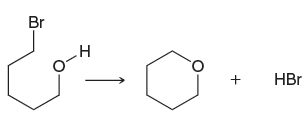Problem: Consider the following reaction: ΔS of this reaction is positive. Explain.

FREE Expert Solution
90% (209 ratings)
Problem Details

Consider the following reaction:

ΔS of this reaction is positive. Explain.What scientific concept do you need to know in order to solve this problem?

Our tutors have indicated that to solve this problem you will need to apply the Entropy concept. You can view video lessons to learn Entropy Or if you need more Entropy practice, you can also practice Entropy practice problems .

What is the difficulty of this problem?

Our tutors rated the difficulty of Consider the following reaction: ΔS of this reaction is pos... as high difficulty.

How long does this problem take to solve?

Our expert Organic tutor, Chris took 2 minutes to solve this problem. You can follow their steps in the video explanation above.

What textbook is this problem found in?

Our data indicates that this problem or a close variation was asked in . You can also practice practice problems .